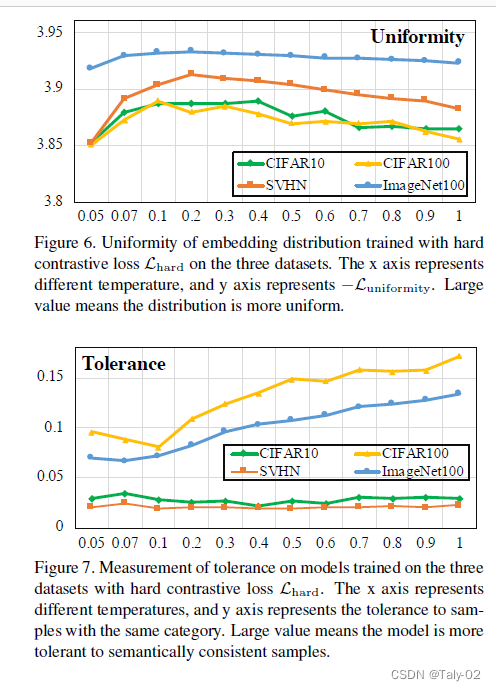# CVPR 2021 如何理解对比学习中的温度系数？

### 2. 引入¶

https://blog.csdn.net/pitaojun/article/details/108869569

• Alignment 让相同的样本的特征尽可能相似 。
• Uniformity使得特征的分布保持尽可能多的信息。# bsz : batch size (number of positive pairs)
# d : latent dim
# x : Tensor, shape=[bsz, d]
# latents for one side of positive pairs
# y : Tensor, shape=[bsz, d]
# latents for the other side of positive pairs
def align_loss(x, y, alpha=2):
return (x - y).norm(p=2, dim=1).pow(alpha).mean()

def uniform_loss(x, t=2):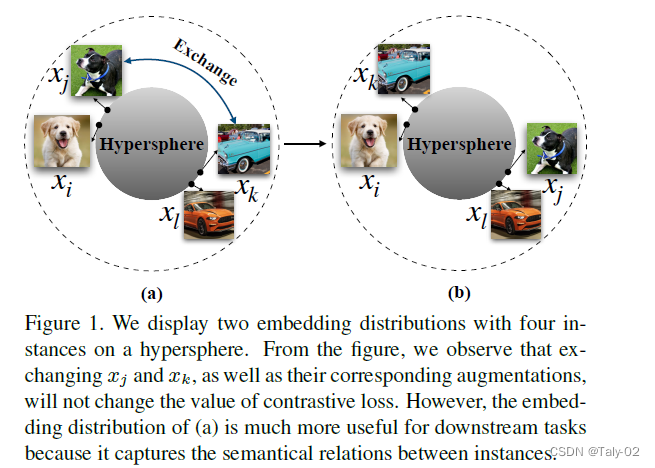### 4. 方法¶

\mathcal{L}\left(x_i\right)=-\log \left[\frac{\exp \left(s_{i, i} / \tau\right)}{\sum_{k \neq i} \exp \left(s_{i, k} / \tau\right)+\exp \left(s_{i, i} / \tau\right)}\right]

\mathcal{L}_{\text {simple }}\left(x_i\right)=-s_{i, i}+\lambda \sum_{i \neq j} s_{i, j}

\frac{\partial \mathcal{L}\left(x_i\right)}{\partial s_{i, i}}=-\frac{1}{\tau} \sum_{k \neq i} P_{i, k}
\frac{\partial \mathcal{L}\left(x_i\right)}{\partial s_{i, j}}=\frac{1}{\tau} P_{i, j}
P_{i, j}=\frac{\exp \left(s_{i, j} / \tau\right)}{\sum_{k \neq i} \exp \left(s_{i, k} / \tau\right)+\exp \left(s_{i, i} / \tau\right)}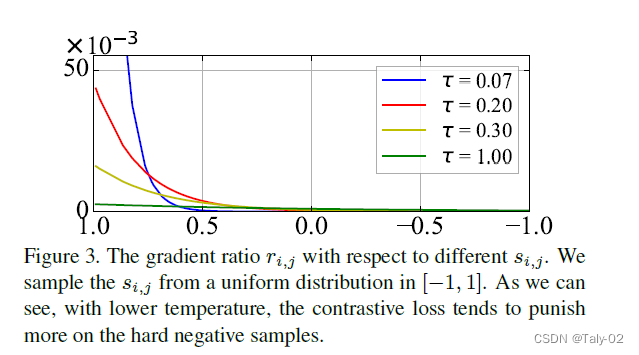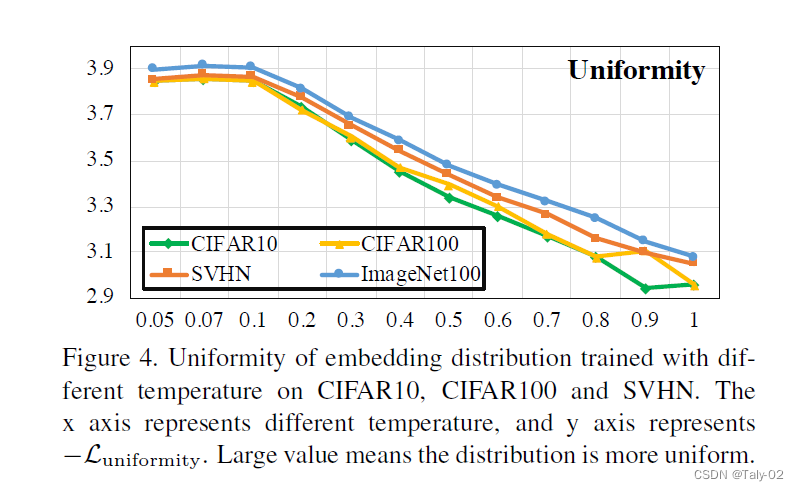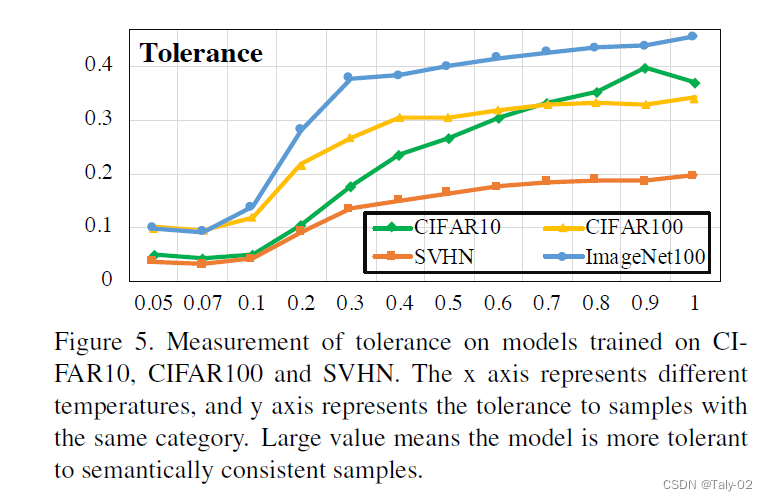\mathcal{L}_{\text {hard }}=-\log \frac{\exp \left(s_{i, i} / \tau\right)}{\sum_{s_{i, k} \geq s_\alpha^{(i)}} \exp \left(s_{i, k} / \tau\right)+\exp \left(s_{i, i} / \tau\right)}